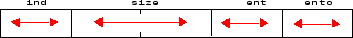# wptree

WPTREE constructor

## Syntax

```T = wptree(ORDER,DEPTH,X,WNAME,ENT_TYPE,PARAMETER) T = wptree(ORDER,DEPTH,X,WNAME) T = wptree(ORDER,DEPTH,X,WNAME,'shannon') T = wptree(ORDER,DEPTH,X,WNAME,ENT_TYPE,ENT_PAR,USERDATA) ```

## Description

`T = wptree(ORDER,DEPTH,X,WNAME,ENT_TYPE,PARAMETER)` returns a complete wavelet packet tree `T`.

`ORDER` is an integer representing the order of the tree (the number of “children” of each non terminal node). `ORDER` must be equal to 2 or 4.

If `ORDER = 2`, `T` is a WPTREE object corresponding to a wavelet packet decomposition of the vector (signal) `X`, at level `DEPTH` with a particular wavelet `WNAME`.

If `ORDER = 4`, `T` is a WPTREE object corresponding to a wavelet packet decomposition of the matrix (image) `X`, at level `DEPTH` with a particular wavelet `WNAME`.

`ENT_TYPE` is a character vector or string scalar containing the entropy type and `ENT_PAR` is an optional parameter used for entropy computation (see `wentropy`, `wpdec`, or `wpdec2` for more information).

`T = wptree(ORDER,DEPTH,X,WNAME)` is equivalent to `T = wptree(ORDER,DEPTH,X,WNAME,'shannon')`

With `T = wptree(ORDER,DEPTH,X,WNAME,ENT_TYPE,ENT_PAR,USERDATA)` you may set a userdata field.

The function `wptree` returns a WPTREE object.

For more information on object fields, see the `get` function or type

```help wptree/get ```

Class WPTREE (Parent class: DTREE)

## Fields

 `'dtree'` DTREE parent object `'wavInfo'` Structure (wavelet information) `'entInfo'` Structure (entropy information)

The wavelet information structure, `'wavInfo'`, contains

 `'wavName'` Wavelet name `'Lo_D'` Low Decomposition filter `'Hi_D'` High Decomposition filter `'Lo_R'` Low Reconstruction filter `'Hi_R'` High Reconstruction filter

The entropy information structure, `'entInfo'`, contains

 `'entName'` Entropy name `'entPar'` Entropy parameter

Fields from the DTREE parent object:

 'allNI' All nodes information

`'allNI'` is an array of size `nbnode` by `5`, which contains

 `ind` Index `size` Size of data `ent` Entropy `ento` Optimal entropy

Each line is built based on the following scheme:## Examples

```% Create a wavelet packet tree. x = rand(1,512); t = wptree(2,3,x,'db3'); t = wpjoin(t,[4;5]); % Plot tree t4. plot(t); % Click the node (3,0), (see the `plot` function). ```